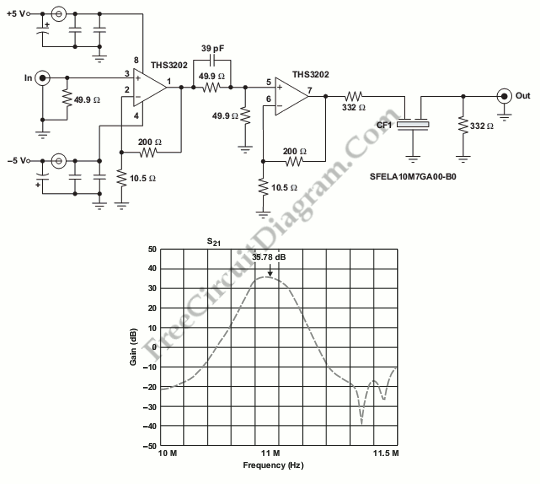# 10.7-MHz IF amplifierThis is 10.7-MHz IF amplifier circuit. This circuit is used in many application such as cell phone base station receivers (final IF) and FM broadcast receivers. The ceramic filter that is used in this circuit are cheap and available in a variety of insertion losses and banwidths. This circuit uses a 230-kHz bandwidth filter SFELA10M7GA00-B0 and the gain stage. Here is the circuit :The second figure shows the circuit response. The insertion loss of this circuit is 4 dB. However, actually the insertion loss is slightly more than 4dB. In the passband, the slight nonlinearity  has more to do with the ceramic filter’s characteristics than with the op amp. The circuit’s impedances are different from a nominal load of 50 Ohm, so the response should be corrected. Actually, a 280-Ohm resistor and the 50-Ohm instrument as the load that is configured in series connection measures the response. The voltage divider in this circuit is used to compesate that effect. [Source: Texas Instruments Application Note]